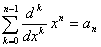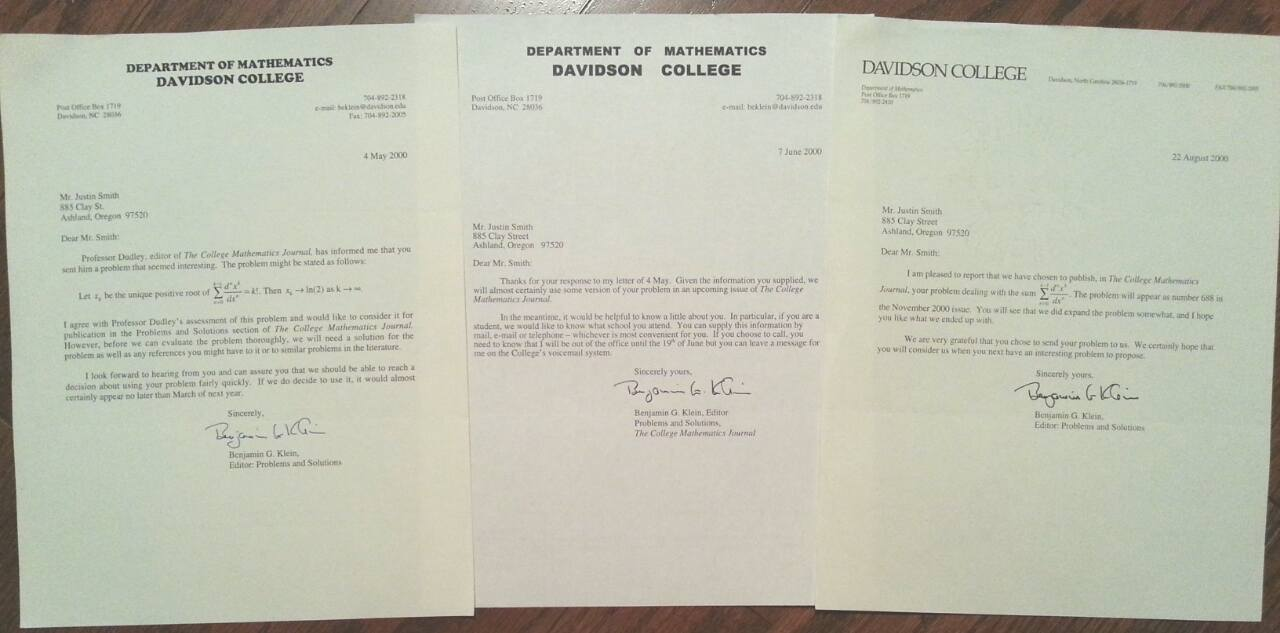If you find any of this useful, please consider donating via PayPal to help keep this site going.# The College Mathematics Journal, Vol. 31, No. 5, Nov. 2000, problem 688, p. 409

11/2000

Let an, n>=1 denote a sequence of positive real numbers, and for each n>=1, define rn to be the unique positive solution to the equationGiven a nonnegative number L, characterize those sequences an such that lim n-->oo an = L.

Hint:

I created the following problem while I was playing around with some graphs. Consider graphing the following equations:

1. y = x2 + 2x, y = 2
2. y = x3 + 3x2 + 6x, y = 6
3. y = x4 + 4x3 + 12x2 + 24x, y = 24
4. y = x5 + 5x4 + 20x3 + 60x2 + 120x, y = 120
etc. (those familiar with differentation of polynomials will notice the pattern)

Notice that the x value of the intersection for each pair of equations decreases. However, if the process were continued, we know the x value of the intersection would not be 0, because we'd have

0 = really large number

which is not possible. Therefore the x value must be greater than 0, but what x value is it?

Here is the correspondence I have on the problem: